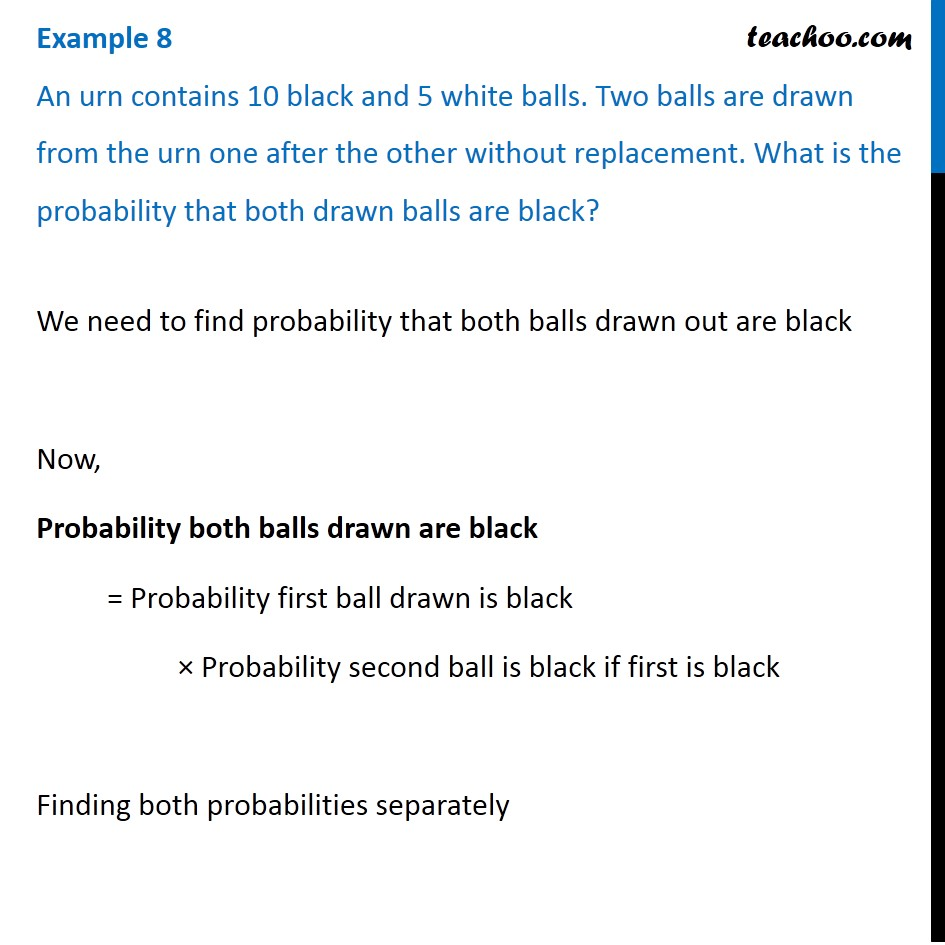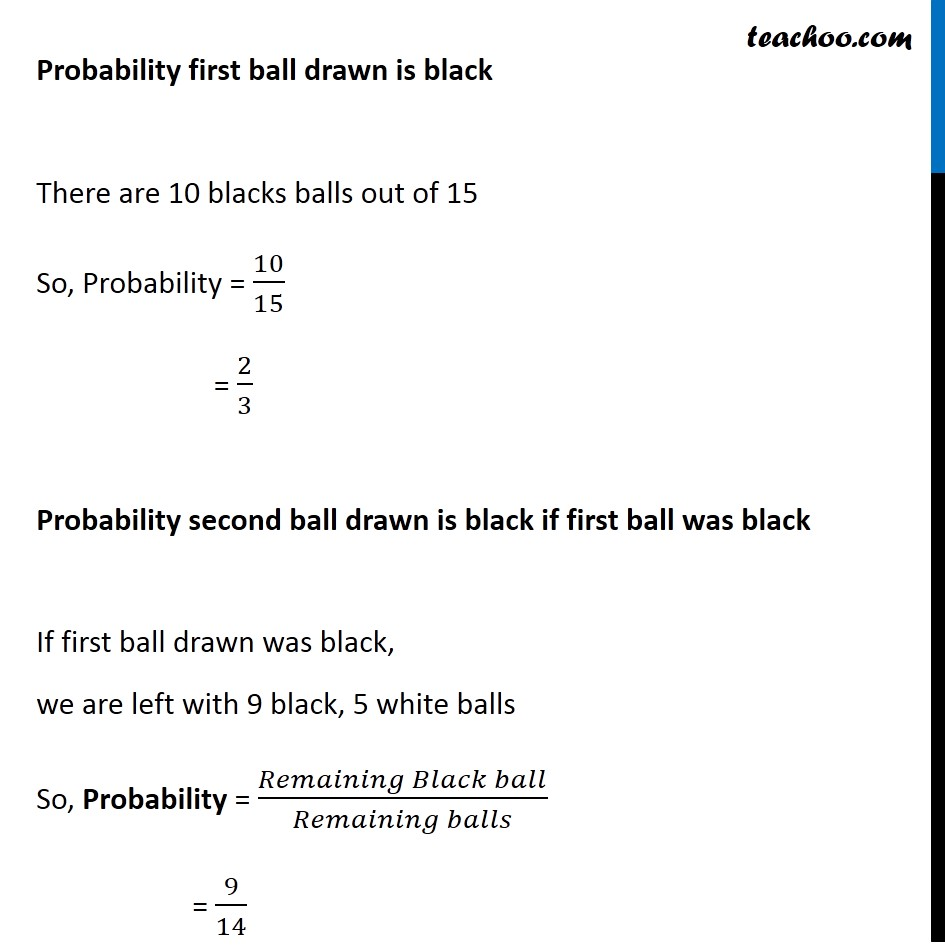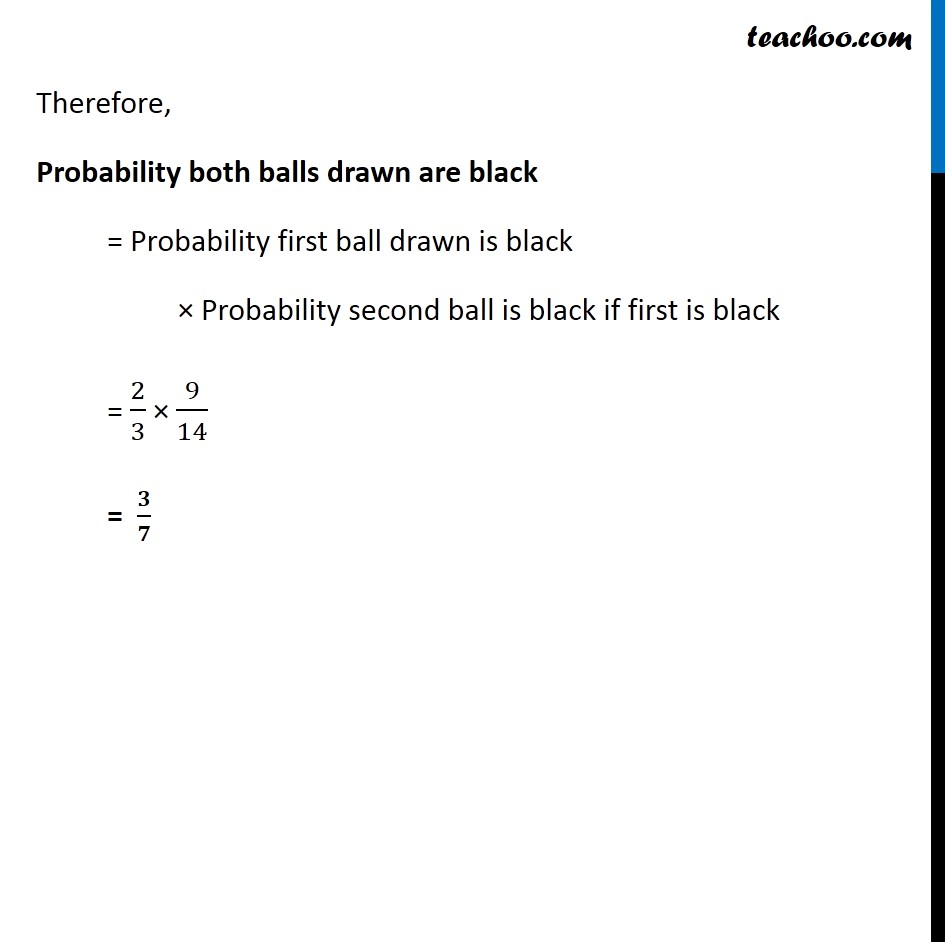Multiplication rule of probability

Chapter 13 Class 12 Probability
Concept wiseSolve all your doubts with Teachoo Black (new monthly pack available now!)

### Transcript

Example 8 An urn contains 10 black and 5 white balls. Two balls are drawn from the urn one after the other without replacement. What is the probability that both drawn balls are black? We need to find probability that both balls drawn out are black Now, Probability both balls drawn are black = Probability first ball drawn is black × Probability second ball is black if first is black Finding both probabilities separately Probability first ball drawn is black There are 10 blacks balls out of 15 So, Probability = 10/15 = 2/3 Probability second ball drawn is black if first ball was black If first ball drawn was black, we are left with 9 black, 5 white balls So, Probability = (𝑅𝑒𝑚𝑎𝑖𝑛𝑖𝑛𝑔 𝐵𝑙𝑎𝑐𝑘 𝑏𝑎𝑙𝑙)/(𝑅𝑒𝑚𝑎𝑖𝑛𝑖𝑛𝑔 𝑏𝑎𝑙𝑙𝑠) = 9/14 Therefore, Probability both balls drawn are black = Probability first ball drawn is black × Probability second ball is black if first is black = 2/3 × 9/14 = 𝟑/𝟕